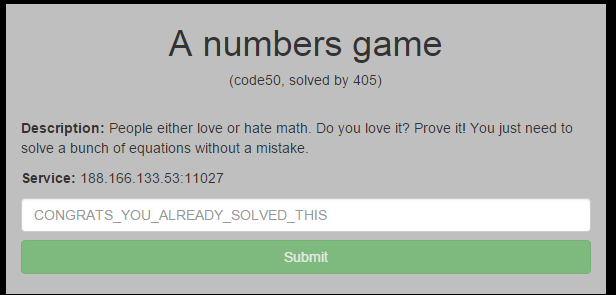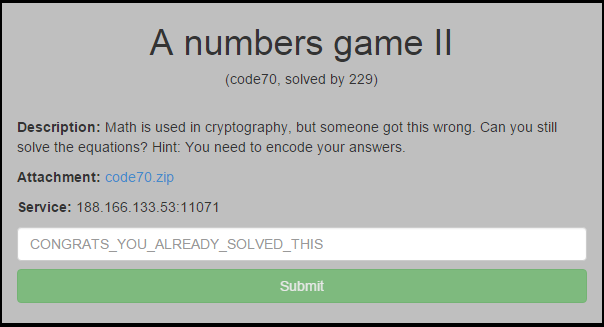By Sam Brown (@_samdb_)

As the names give away these two were linked so I've written them up together. Advanced apologies from the code here, it's pretty disgusting.

##### A Numbers GameWhen we connect to the service it gives us a basic equation to solve for x and asks for the answer, I spent a bunch of time trying to use sympy to solve this the smart way but after failing for a while I gave up and fell back on gold fashioned brute forcing and running 'eval()' on data from a strange server...

```import socket

def get_x(equa):
equa = equa.replace('=','==')
for i in range(-10000,10000):
out = eval(equa.replace('x',str(i)))
if out != False:
print equa
print 'x = ' + str(i)
return i

if __name__ == "__main__":
s = socket.socket(socket.AF_INET, socket.SOCK_STREAM)
s.connect(("188.166.133.53", 11027))
while True:
data = s.recv(1024)
while data.find(':') == -1:
print data
data = s.recv(1024)
start = data.find(':') + 2
print data
num = get_x(data[start:])
print num
s.send(str(num) + "\n")
```

Not my proudest moment but after solving 100 equations out popped the flag.

##### A Numbers Game 2Inside the zip we get the following code:

```This snippet may help:

def encode(self, eq):
out = []
for c in eq:
q = bin(self._xor(ord(c),(2<<4))).lstrip("0b")
q = "0" * ((2<<2)-len(q)) + q
out.append(q)
b = ''.join(out)
pr = []
for x in range(0,len(b),2):
c = chr(int(b[x:x+2],2)+51)
pr.append(c)
s = '.'.join(pr)
return s
```

This time when we connect to the service we get mostly the same information but the equation has been encoded in some way, it was safe to assume it was with the function they gave us so I started off by writing a decoder. Adding some logging to get the value of b when encoding something I found that the first loop was turning an input string into a binary string with each input character being represented by 2 characters in the string. This string is then turned into the output numbers seperated by dots by the following loop, the following code does the inverse of these operations.

```def decode(eq):
out = ''
pr = eq.split('.')
tmp = []
for i in pr:
b = bin(ord(i) - 51).lstrip("0b")
tmp.append(("0" * (2 - len(b))) + str(b))
tmp = ''.join(tmp)
chars = [tmp[i:i+8] for i in range(0, len(tmp), 8)]
for i in chars:
out += chr(int(i,2) ^ 2<<4)
return out
```

Combining this with the code from the previous challenge was successful and after another 100 equations, out popped the flag.

```import socket
def xor(a,b):
return a ^ b

def encode(eq):
print eq
out = []
for c in eq:
q = bin(xor(ord(c),(2<<4))).lstrip("0b")
print q
q = "0" * ((2<<2)-len(q)) + q
out.append(q)
b = ''.join(out)
print b
pr = []
for x in range(0,len(b),2):
c = chr(int(b[x:x+2],2)+51)
pr.append(c)
s = '.'.join(pr)
print s
return s

def decode(eq):
out = ''
pr = eq.split('.')
tmp = []
for i in pr:
b = bin(ord(i) - 51).lstrip("0b")
tmp.append(("0" * (2 - len(b))) + str(b))
tmp = ''.join(tmp)
chars = [tmp[i:i+8] for i in range(0, len(tmp), 8)]
for i in chars:
out += chr(int(i,2) ^ 2<<4)
return out

def get_x(equa):
equa = equa.replace('=','==')
for i in range(-10000,10000):
out = eval(equa.replace('x',str(i)))
if out != False:
print equa
print 'x = ' + str(i)
return i

if __name__ == "__main__":
s = socket.socket(socket.AF_INET, socket.SOCK_STREAM)
s.connect(("188.166.133.53", 11071))
while True:
data = s.recv(1024)
print data
if data != '':
line = data.split('\n')
for l in line:
if l.find(':') != -1:
parsed = l[l.find(':') + 2:]
print parsed
eq = decode(parsed)
print eq
x = get_x(eq)
print x
s.send(encode(str(x)) + '\n')
```